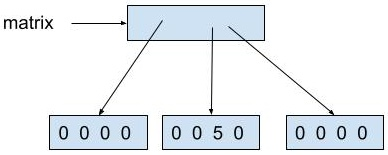# Intro to 2D arrays

Consider the 2D array of `int` values below.

``````int[][] matrix = new int;
matrix = 5;
``````

The 2D array can be thought of as a grid of rows and columns or as an array of 1D arrays.

## As a grid of rows and columns

The array above can be thought of as a grid of 3 rows and 4 columns.

``````   0 1 2 3
0: 0 0 0 0
1: 0 0 5 0
2: 0 0 0 0
``````

The element at row `1` column `2` is `5`. As with all arrays of primitive numeric type, all other values are `0`.

In many problems that use 2D arrays, it is advantageous to visualize the 2D array as a grid of rows and columns.

It is possible to create multidimensional arrays in Java; however, this is less common. Arrays with more than 2 dimensions are harder to visualize. There is often a better way to represent the same data, such as with an array of objects. The AP CS A Exam features only 1D and 2D arrays.

It is possible to create ragged 2D arrays in Java. In a ragged 2D array the rows may vary in length. This makes visualization and some code a bit more challenging. On the AP CS A Exam, each row in a 2D array will have the same length.

## As an array of 1D arrays

The same array can be thought of as an array of three 1D arrays, each of length 4.

``````[[0 0 0 0] [0 0 5 0] [0 0 0 0]]
``````

In the 1D array at index `1` the element at index `2` is `5`.

Java stores 2D arrays as arrays of 1D arrays. More precisely, Java stores a 2D array as a 1D array with references to (memory addresses of) 1D arrays.See the Arrays as objects exercises for more details of how arrays are treated in Java.

In many problems that use 2D arrays, it is advantageous to think about and work with a 2D array as an array of 1D arrays. On the AP CS A Exam, students are required to be able to access individual rows of a 2D array and treat them as 1D arrays. For example, students could be required to pass each row of a 2D array to a method that accepts a 1D array.

See Treating a 2D array as an array of 1D arrays for a demonstration of calling a method with each row of a 2D array.

## Accessing dimensions

The number of rows (the number of 1D arrays) is accessed as:
`matrix.length`

The number of columns (the length of each 1D array) is accessed as:
`matrix.length`

The array at the top of the page has 3 rows and 4 columns.

## Accessing elements

As described above, `matrix` refers to the element at row `1` column `2`.

`matrix` refers to the entire first row. More precisely, `matrix` stores the memory address of the 1D array representing the first row.